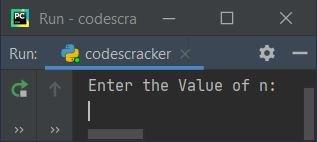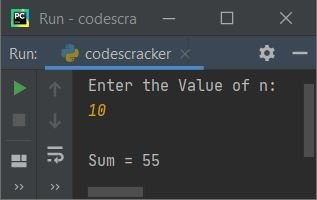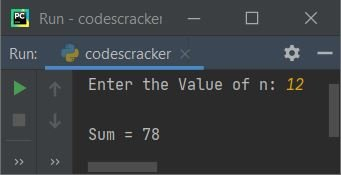# Python Program to Find Sum of n Natural Numbers

This article is created to cover some programs in Python, that find and prints sum of n natural numbers. The value of n must be entered by user at run-time. Here are the list of approaches used:

• Find sum of n natural number using while loop
• Using for loop
• Using function
• Using class

Note - Sum of first 10 natural numbers is calculated as 1+2+3+4+5+6+7+8+9+10, that is equal to 55.

## Find Sum of n Natural Numbers using while Loop

The question is, write a Python program to find sum of n natural numbers.. If user enters 10 as value of n then program will find and print sum of first 10 natural numbers like shown in the program given below:

```print("Enter the Value of n: ")
n = int(input())

sum = 0
i = 1
while i<=n:
sum = sum+i
i = i+1

print("\nSum =", sum)```

Here is the initial output produced by this Python program:Now supply the input say 10 as value of n to find and print sum of first 10 natural numbers like shown in the snapshot given below:The dry run of above program with user input 10 goes like:

• Initial values, n=10 (entered by user), sum=0, i=1
• Now the condition of while loop, i<=n gets evaluated
• That is, the condition i<=n or 1<=10 evaluates to be true, therefore program flow goes inside the loop and sum+i or 0+1 or 1 gets initialized to sum. So sum=1
• And i+1 or 1+1 or 2 gets initialized to i. So i=2
• Now program flow again evaluates the condition of while loop. That is, the condition i<=n or 2<=10 evaluates to be true, therefore program flow again goes inside the loop. This process continues until the condition evaluates to be false
• Therefore after exiting from the loop, the variable sum holds the result value. Print the value of it

## Sum of n Natural Numbers using for Loop

This program is created using for loop. The try-except is used to handle with invalid inputs. The end used here to skip inserting an automatic newline.

```print("Enter the Value of n: ", end="")
try:
n = int(input())
if n<0:
print("\nInvalid Input!")
else:
sum = 0
for i in range(1, n+1):
sum = sum+i
print("\nSum =", sum)
except ValueError:
print("\nInvalid Input!")```

Here is its sample run with 12 as user input:The following code (from above program):

`for i in range(1, n+1):`

is used to execute the following statement:

`sum = sum+i`

n number of times with value of i from 1 to n. The range() method used here, returns a sequence of values starting from a value 1 (provided as its first argument) to (n+1)-1 or n (provided as its second argument).

## Sum of n Natural Numbers using Function

This program is created using a user-defined function named SumOfN(). That is, using the statement, sum = SumOfN(n), the function SumOfN() gets called with its argument value as n. That is, n gets passed as argument of SumOfN() function.

Therefore n's value gets copied to k and using k, the sum of first k natural numbers gets calculated and initialized to s. And s's value gets returned, so its value gets initialized to sum. And the value of sum gets printed as output.

```def SumOfN(k):
s = 0
for i in range(1, k+1):
s += i
return s

print("Enter the Value of n: ", end="")
try:
n = int(input())
if n<0:
print("\nInvalid Input!")
else:
sum = SumOfN(n)
print("\nSum =", sum)
except ValueError:
print("\nInvalid Input!")```

## Sum of n Natural Numbers using Class

This is the last program of this article, created using a class named CodesCracker. Inside this class, I've created a member function named SumOfN(). This function does the same job as of previous program's function.

The only difference is, it is created inside a class, therefore to access it, we've to create an object of this class and then can access it using its object with dot (.) operator like shown in the program given below:

```class CodesCracker:
def SumOfN(self, k):
s = 0
for i in range(1, k+1):
s += i
return s

print("Enter the Value of n: ", end="")
n = int(input())
if n<0:
print("\nInvalid Input!")
else:
ob = CodesCracker()
print("\nSum =", ob.SumOfN(n))```

Python Online Test

« Previous Program Next Program »